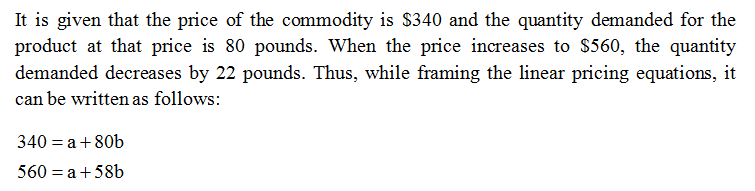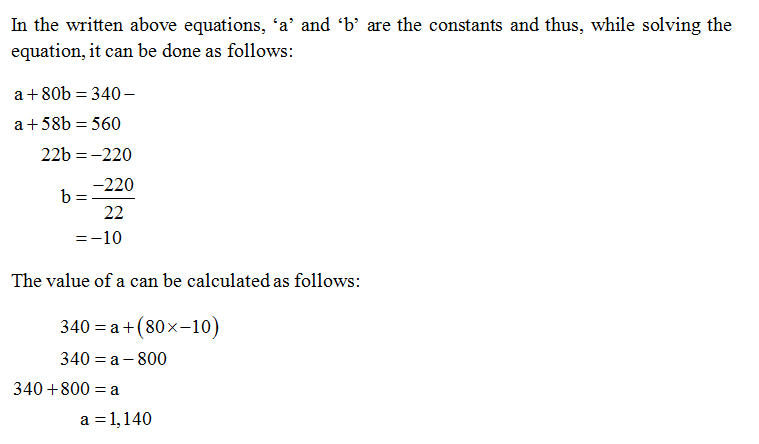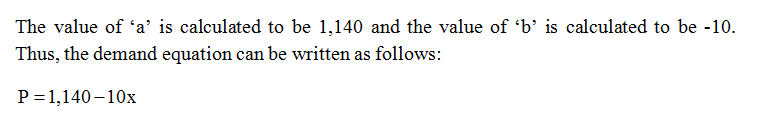# At a unit price of \$340, the quantity demanded of a certain commodity is 80 pounds. If the unit price increases to \$560, the quantity demanded decreases by 22 pounds. Find the demand equation (assuming it is linear) where p is the unit price and x is the quantity demanded for this commodity in pounds.p=At what price are no consumers willing to buy this commodity? According to the above model, how many pounds of this commodity would consumers take if it was free?

Question
4 views

At a unit price of \$340, the quantity demanded of a certain commodity is 80 pounds. If the unit price increases to \$560, the quantity demanded decreases by 22 pounds. Find the demand equation (assuming it is linear) where p is the unit price and x is the quantity demanded for this commodity in pounds.

p=

At what price are no consumers willing to buy this commodity?

According to the above model, how many pounds of this commodity would consumers take if it was free?

check_circle

Step 1Step 2Step 3...

### Want to see the full answer?

See Solution

#### Want to see this answer and more?

Solutions are written by subject experts who are available 24/7. Questions are typically answered within 1 hour.*

See Solution
*Response times may vary by subject and question.
Tagged in